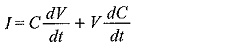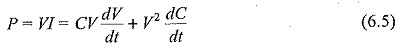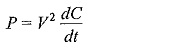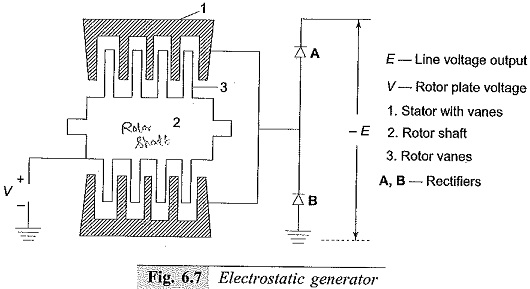## Electrostatic Generators:

Van de Graaff generators are essentially high voltage but low power devices, and their power rating seldom exceeds few tens of kilowatts. As such Electrostatic Generators machine which effectively convert mechanical energy into electrical energy using variable capacitor principle were developed. These are essentially duals of electromagnetic machines and are constant voltage variable capacitance machines.

An Electrostatic Generators consists of a stator with interleaved rotor vanes forming a variable capacitor and operates in vaccum.

The current through a variable capacitor is given bywhere

C is a capacitor charged to a potential V.

The power input into the circuit at any instant isIf dc/dt is negative, mechanical energy is converted into electrical energy.

With the capacitor charged with a d.c. voltage V, C dv/dt  = 0 and the power output will beA schematic diagram of a synchronous electrostatic generator with interleaved stator and rotor plates is shown in Fig. 6.7. The rotor is insulated from the ground, and is maintained at a potential of + V. The rotor to stator capacitance varies from C0 to Cm, and the stator is connected to a common point between two rectifiers across the d.c. Output which is -E volts.

When the capacitance of the rotor is maximum (Cm), the rectifier B does not conduct and the stator is at ground potential. The potential E is applied across the rectifier A and V is applied across Cm. As the rotor rotates, the capacitance C decrease and the voltage across C increases.Thus, the stator becomes more negative with respect to ground. When the stator reaches the line potential – E the rectifier A conducts, and further movement of the rotor causes the current to flow from the generator. Rectifier B will now have E across it and the charge left in the generator will be Q0 = C(V + E) + E(Cs + Cr), where Cs is the stator capacitance to earth, Cr is the capacitance of rectifier B to earth, and C0 is the minimum capacitance value of C (stator to rotor capacitance).

A generator of this type with an output voltage of one MV and a field gradient of 1 MV/cm in high vacuum and having 16 rotor poles, 50 rotor plates of 4 feet maximum and 2 feet minimum diameter, and at a speed of 4000 rpm would develop 7 MW of power.

Scroll to Top